Question

# Calculate the change in the internal energy of the system for a process in which the...

Calculate the change in the internal energy of the system for a process in which the system absorbs 140 J of heat from the surroundings and does 85 J of work on the surroundings.

Dear student,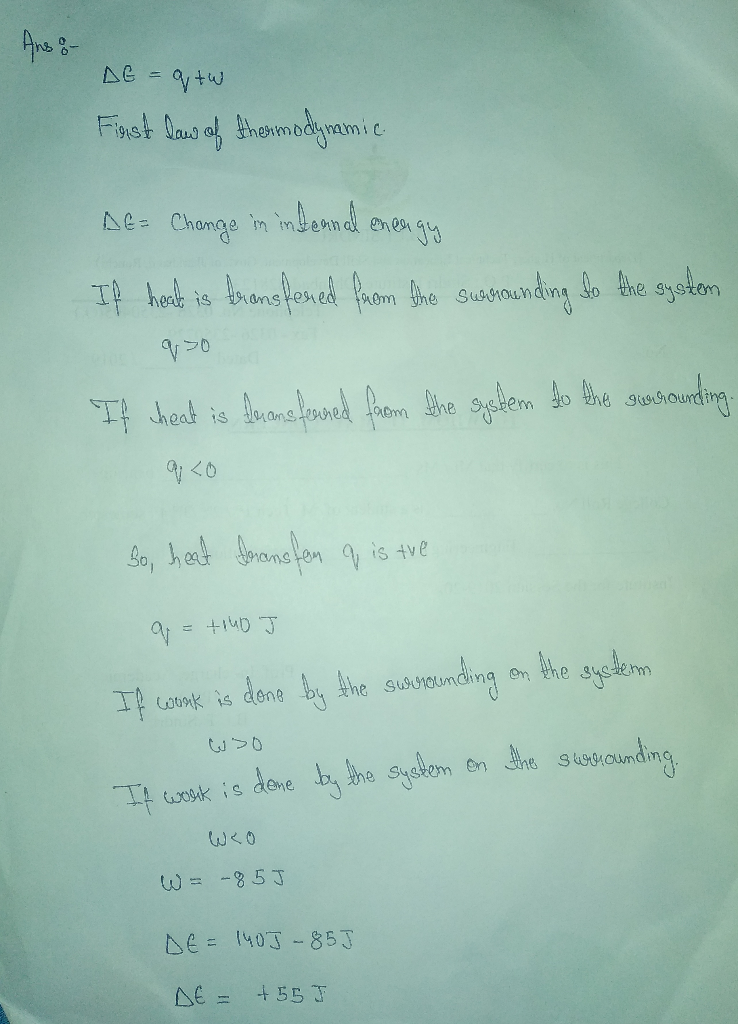With regards....

#### Earn Coins

Coins can be redeemed for fabulous gifts.

Similar Homework Help Questions
• ### 2. Work and Heat. Calculate the change in the internal energy of the system for a...2. Work and Heat. Calculate the change in the internal energy of the system for a process in which the system absorbs 140 J of heat from the surroundings and does 85 J of work on the surroundings,

• ### 1) For the following processes, calculate the change in internal energy of the system and...

1) For the following processes, calculate the change in internal energy of the system and determine whether the process is endothermic or exothermic: (a) A balloon iscooled by removing 0.655 kj of heat. It shrinks on cooling, and the atmosphere does 382 j of work on the balloon. (b) A 100.0-g bar of gold is heated from 25°C to 50°Cduring which it absorbs 322 j of heat. Assume the volume of the gold bar remains constant. (c) the surroundings do...

• ### 400 J of heat is added to a system. If the change in internal energy of...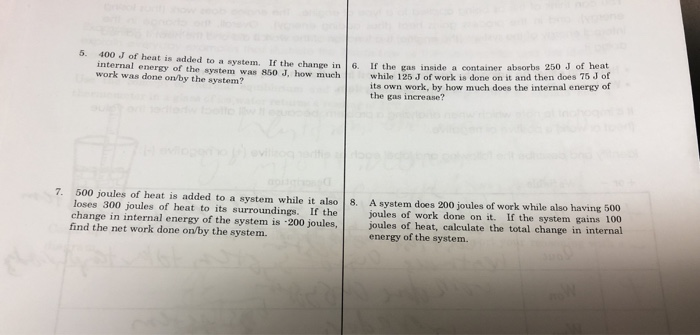400 J of heat is added to a system. If the change in internal energy of the system was 850 J, how much work was done on by the system? the inside a container absorbs 250 J of hea while 125 J of work is done on it and then does 70 J of its own work, by how much does the internal energy of the gas increase? 7.500 joules of heat is added to a system while it also...

• ### is this correct? Determine the change in internal energy (AE) of a system that absorbs 745...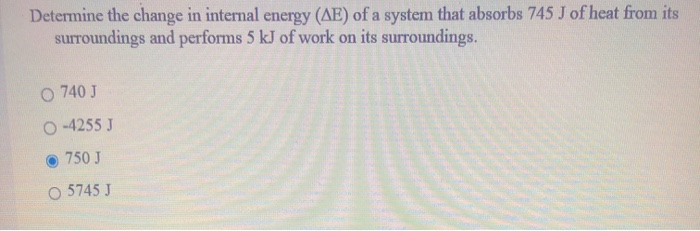is this correct? Determine the change in internal energy (AE) of a system that absorbs 745 J of heat from its surroundings and performs 5 kJ of work on its surroundings. O 740 J O-4255 J 750J o 5745 )

• ### Determine the change in internal energy (AE) of a system that absorbs 745 J of heat...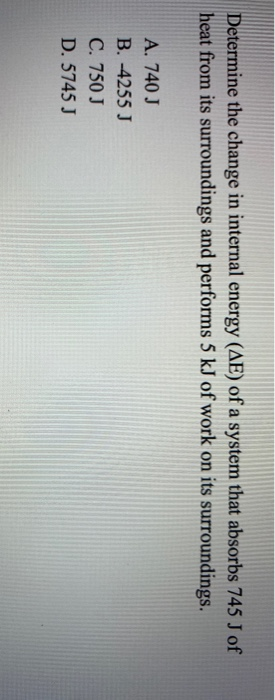Determine the change in internal energy (AE) of a system that absorbs 745 J of heat from its surroundings and performs 5 kJ of work on its surroundings. A. 740 J B. -4255 J C. 750 J D. 5745 J

• ### 1. Calculate the change in the internal energy of the following systems and then determine whether...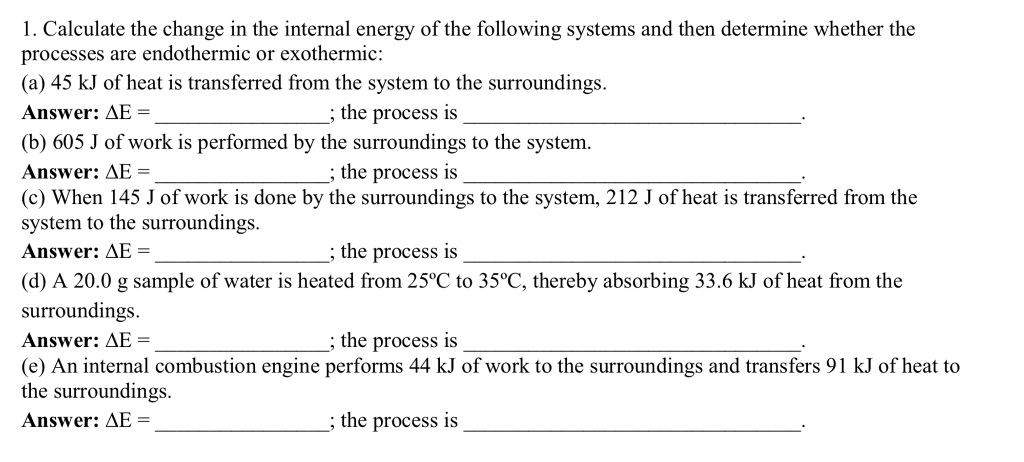1. Calculate the change in the internal energy of the following systems and then determine whether the processes are endothermic or exothermic: (a) 45 kJ of heat is transferred from the system to the surroundings. Answer: AE- the process is (b) 605 J of work is performed by the surroundings to the system. Answer: AE (c) When 145 J of work is done by the surroundings to the system, 212 J of heat is transferred from the system to the...

• ### What is the change in internal energy of a system if the system (i) absorbs 58...

What is the change in internal energy of a system if the system (i) absorbs 58 J of heat and does 58 J of work? (ii) absorbs 125 J of heat and does 687 J of work? (iii) evolves 280 cal of heat and has 1.25 kJ of work done on it?

• ### What is the change in internal energy (in J) of a system that absorbs 0.297 kJ...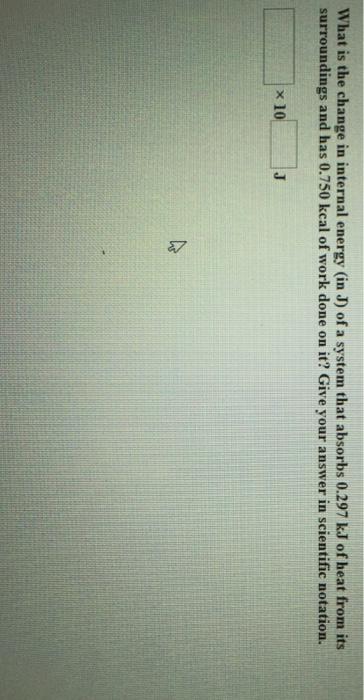What is the change in internal energy (in J) of a system that absorbs 0.297 kJ of heat from its surroundings and has 0.750 kcal of work done on it? Give your answer in scientific notation. × 10,

• ### 1. A gas absorbs 0.0 J of heat and then performs 30.8 J of work. The change in internal energy of the gas is a. 61....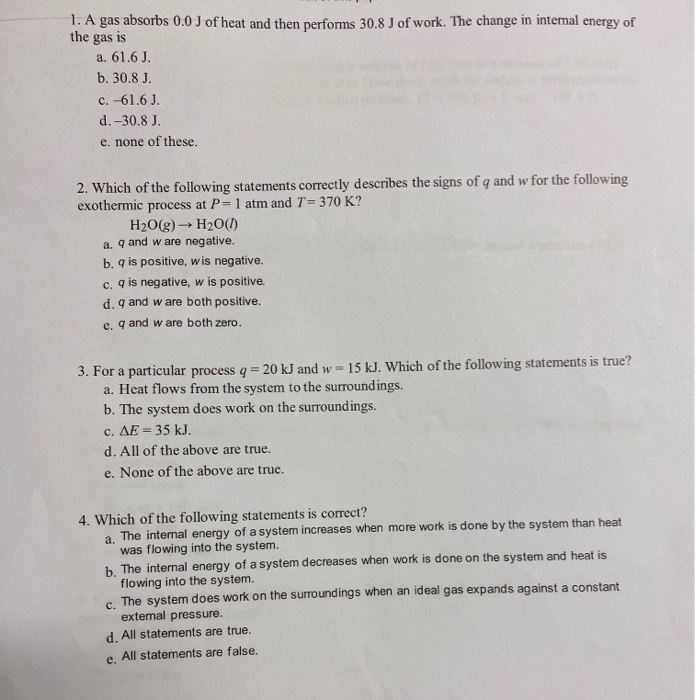1. A gas absorbs 0.0 J of heat and then performs 30.8 J of work. The change in internal energy of the gas is a. 61.6 J. b. 30.8 J. c. -61.6 J. d.-30.8 J. e. none of these 2. Which of the following statements correctly describes the signs of q and w for the following exothermic process at P= 1 atm and T = 370 K? H2O(g) → H2O(1) a. q and w are negative. b. is positive, wis...

• ### Calculate the change in total internal energy for a system that releases 2.84 × 104 kJ...

Calculate the change in total internal energy for a system that releases 2.84 × 104 kJ of heat and does 6.76 × 104 kJ of work on the surroundings. Enter your answer in scientific notation.